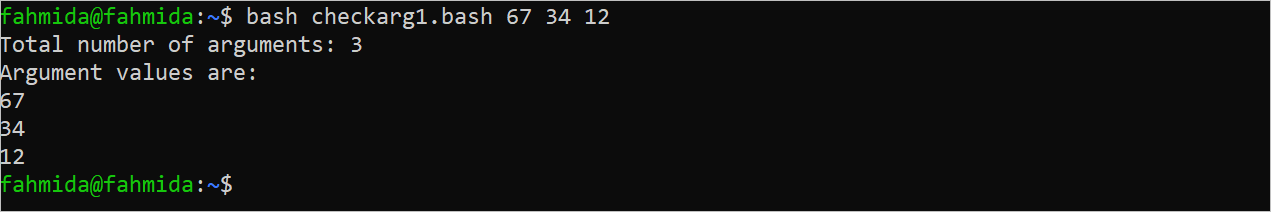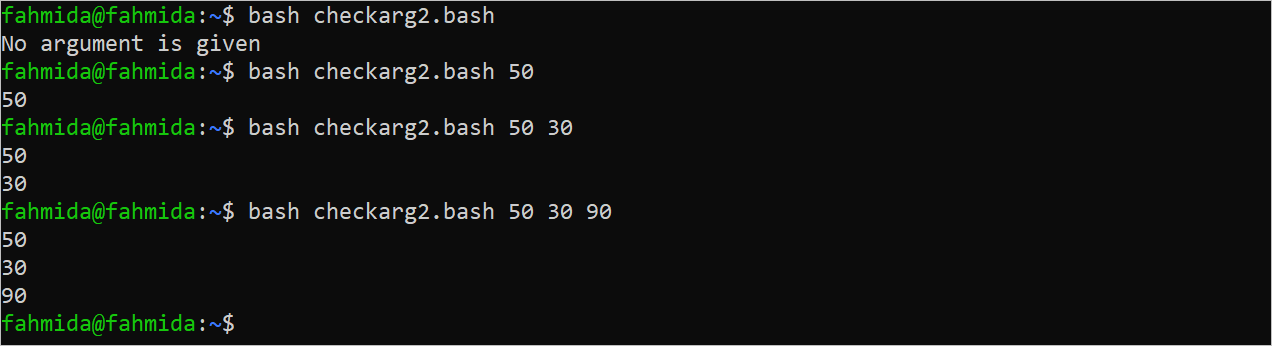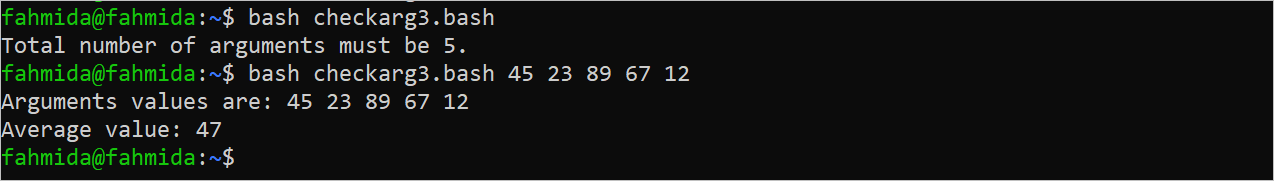| by Arround The Web | No comments

## Check the Number of Arguments in the Bash Script

It is essential to count the total number of arguments that are passed to the script for various purposes such as error handling, providing messages based on the number of arguments, and helping the user to pass the correct number of arguments. The total number of arguments can be counted in Bash in two ways. One is using “\$#” and another by using a loop. The methods of checking the number of arguments and using this value for different purposes are shown in this tutorial.

## Different Uses of Checking the Number of Arguments

The uses of checking the number of arguments are shown in this part of the tutorial using multiple examples.

### Example 1: Count the Total Number of Arguments Using “\$#”

Create a Bash file with the following script that counts the total number of arguments and print the argument values using a “for” loop.

#!/bin/bash

#Store the number of arguments

len=\$#

echo "Total number of arguments: \$len"

echo "Argument values are:"

#Print the argument values

for val in \$@

do

echo \$val

done

The following output appears after executing the script with the argument values of 67, 34, and 12:### Example 2: Print the Argument Values Based on the Argument Length

Create a Bash file with the following script that counts the total number of arguments and print the argument values based on the number of arguments. An error message is printed if no argument is passed to the script.

#!/bin/bash

#Store the number of arguments

len=\$#

#check the total number of arguments

if [ \$len -eq 0 ]; then

echo "No argument is given"

fi

#initialize the counter

counter=0

#Print argument value based on the counter value

while (( \$counter < \$len ))

do

if [ \$counter -lt 1 ]; then

echo \$1

elif [ \$counter -lt 2 ]; then

echo \$2

elif [ \$counter -lt 3 ]; then

echo \$3

fi

((counter++))

done

The script is executed four times in the output. The error message is printed when no argument is given. The argument values are printed when one, two, and three argument values are given.### Example 3: Calculate the Average of the Argument Values

Create a Bash file with the following script that counts the total number of arguments and print the average value of five argument values. The “bc” command is used in the script to calculate the average value. An error message is printed if no argument is passed to the script.

#!/bin/bash

#Check the total number of arguments

if [ \$# -eq 5 ]; then

#Calculate the sum of the argument values

sum=\$((\$1+\$2+\$3+\$4+\$5))

#Calculate the average values

avg=\$((\$sum/5 | bc -l))

#Print the average values and the argument values

echo "Arguments values are: \$1 \$2 \$3 \$4 \$5"

echo "Average value: \$avg"

else

#Print error message

echo "Total number of arguments must be 5."

fi

The script is executed twice in the output. The error message is printed when no argument is given. The average of the argument values are printed when five argument values are given.### Example 4: Print the Error Message Based on the Argument Values

Create a Bash file with the following script that prints any of the three messages based on the “if” condition. The first “if” condition checks whether the number of arguments is 2 or not. The second “if” condition checks whether the length of the argument value is less than 5 or not. The third “if” condition checks whether the second argument is positive or not.

#!/bin/bash

name=\$1

price=\$2

#Count the length of the second argument

len=\${#name}

#Check the total number of arguments

if [ \$# -ne 2 ]; then

echo "Total number of arguments must be 2."

exit

#Check the length of the first argument

elif [ \$len -lt 5 ]; then

echo "Product name must be minimum 5 characters long."

exit

#Check the value of the second argument

elif [ \$2 -lt 0 ]; then

echo "The price value must be positive."

exit

fi

#Print the argument values

echo "The price of \$name is TK. \$price"

The script is executed four times in the output. The error message, “Total number of arguments must be 2”, is printed when no argument is given. The error message, “Product name must be minimum 5 characters long”, is printed when the length of the first argument is less than five. The error message, “The price value must be positive”, is printed when the second argument is negative.## Conclusion

The uses of the number of arguments in the Bash script for various purposes are shown in this tutorial using multiple examples to help the new Bash users.

Source: linuxhint.com